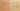RecursiveMethodNeedsIfElse

Misconception:

If a method does not have an if-else statement, then it cannot have a base case and a recursive case, and thus it cannot properly implement a recursive computation that can terminate at some point (by hitting the base case).Incorrect

A recursive method needs to contain an if-else statement

Correct

A recursive method does not necessarily need to contain an if-else statement

CorrectionHere is what's right.

Sometimes a simple if statement, without an else, can be enough:

public void remove(final int index) {
if (tail == null) {
// do nothing
} else {
tail = tail.remove(0, index);
}
}

The above code can (probably better) be expressed as:

public void remove(final int index) {
if (tail != null) {
tail = tail.remove(0, index);
}
}

This kind of single-branch if statement in a recursive method is common when the computation involves side effects (is not a pure function) and the base case means “no action”.

Another situation where no if-else statement is needed is if the recursive call is polymorphic, and one of the possible call targets corresponds to the base case:

interface List {
boolean contains(int value);
}

// base case
class Nil implements List {
boolean contains(int value) {
return false;
}
}

// recursive case
class Cons implements List {
private int value;
private List tail;
public Cons(int value, List tail) {
this.value = value;
this.tail = tail;
}
boolean contains(int value) {
return this.value == value || tail.contains(value); // recursive
}
}

The recursive call site represents a dynamic dispatch, either to Nil.contains() (base case) or to Cons.contains() (recursive case).

OriginWhere could this misconception come from?

This misconception might just be students inappropriately refining the “mantra” “you need a base case and a recursive case” to “you need an if branch and an else branch”.

However, it could also be that this misconception is more about if statements than about recursion. For example, if students previously learned functional programming with a textbook like HtDP, then they are used to pure functions built from expressions with if or cond. And such conditional expressions have to explicitly cover all cases (e.g., there must always be an else case in the if).

ValueHow can you build on this misconception?

Students who hold this misconceptions seem to have internalized that one does need a base case and a recursive case to properly implement a finite recursive computation.

This is valuable!

Java